cancel
Showing results for
Did you mean:Helper I

## matrix Removing Row totals at higher hierarchy and displaying Grand total

Hello All,
I am trying to customize a matrix visualization. this is my excpected Out put how can i achieve this ( Pictures atached).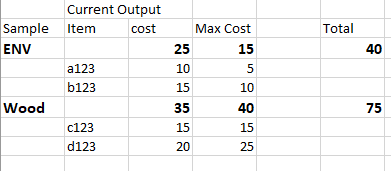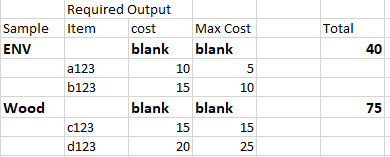1 ACCEPTED SOLUTION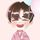Community Support

``Measure = IF(ISINSCOPE('Table'[Item]) ,SUM('Table'[cost]), IF(ISINSCOPE('Table'[Sample]),BLANK(),SUM('Table'[cost])))``

Output: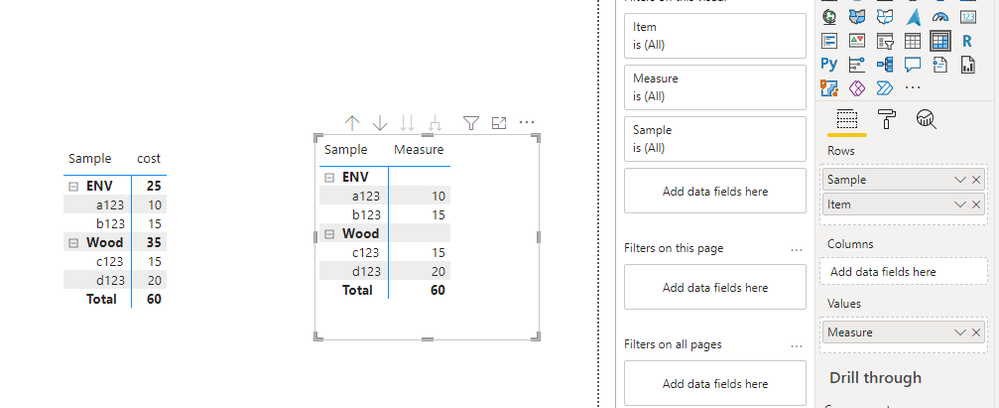Best Regards,
Eyelyn Qin
If this post helps, then please consider Accept it as the solution to help the other members find it more quickly.

5 REPLIES 5Community Support

``Measure = IF(ISINSCOPE('Table'[Item]) ,SUM('Table'[cost]), IF(ISINSCOPE('Table'[Sample]),BLANK(),SUM('Table'[cost])))``

Output:Best Regards,
Eyelyn Qin
If this post helps, then please consider Accept it as the solution to help the other members find it more quickly.Super User

Hi,

This can be achieved by using ISFILTERED combined with IF.

My example data: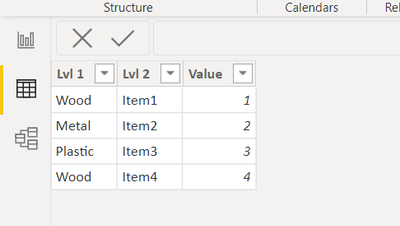The DAX I used:Matrix with Blanks on lvl 1 = IF(
ISFILTERED('Matrix example'[Lvl 1])&&ISFILTERED('Matrix example'[Lvl 2])=FALSE(),"", //This checks if the current row is level 1 if so -> returns blank else returns the calculation below
SUM('Matrix example'[Value])) //Sum can be replaced with measure

End result:Hopefully this helps and if it does consider accepting this as a solution!

Proud to be a Super User!Helper I

Hello,
Thank you for your solution. WHen I have tried this I was able to show Blank values at Level 1 But my Row total per row (Left to right) is also showing as Blanck. I need to show value of the total in there as well. Like using SUMX ???Super User

Hi,

To solve this you can create separate total measure which doesn't have the ISFILTERED structure. So for example something like:

[Total cost]=sum([cost])+sum([Max cost]). Then If you place this Into The Matrix the value will show.

Example: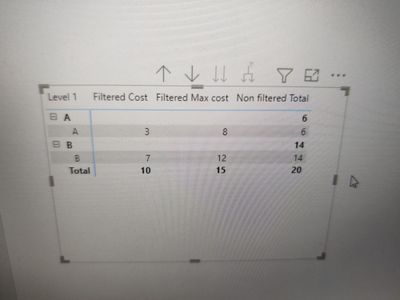Proud to be a Super User!Super User

@dhanekula1996 , I doubt this is possible with standard visual,

As a work around. You need to append data sample distinct values in the item table and the use option Show Item with no data.